# Holonomy group

(diff) ← Older revision | Latest revision (diff) | Newer revision → (diff)

A characteristic of a connection on a fibre bundle. The holonomy group is defined for a principal fibre bundlewith a Lie structure groupand (second countable) baseon which an infinitesimal connectionis given. It is also defined for any fibre bundleassociated towhose fibres are copies of some representation spaceof.

The connectionon(or, respectively, on) defines, for any piecewise-smooth curvein, an isomorphic mappingbetween the fibres corresponding to the beginning and the end of. To each piecewise-smooth closed curveinbeginning and ending at a point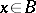corresponds an automorphism of the fibre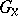(or, respectively,) over the point. These automorphisms form a Lie group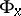, which is called the holonomy group of the connectionat.

If the base is (pathwise) connected, thenandare isomorphic for anyandin. One may accordingly speak of the holonomy group of a bundle(or) with connectionand with (pathwise) connected base.

The holonomy groupis a subgroup of the structure group. In the case of a linear connection onthis subgroup may be defined directly. Let a point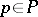in the fibreover a pointbe given. The set of elements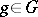such that the pointsand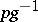can be connected by horizontal curves informs a subgroupof, which is isomorphic to.

The limited (restricted) holonomy group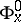is the subgroup of the holonomy groupgenerated by the closed curves that are homotopic to zero. It coincides with the pathwise-connected component of the unit element of; moreover,is at most countable.

The role of holonomy groups in the differential geometry of fibre bundles is explained by the following theorems on connections on.

Reduction theorem. Letbe a principal fibre bundle satisfying the second axiom of countability; letbe the holonomy group of the connectiondefined on. Then the structure groupis reducible to its subgroup, and the connectionis reducible to a connection on the reduced fibre bundle, the holonomy group of which coincides with.

Holonomy theorem. The holonomy algebra (the algebra of the restricted holonomy group) is a subalgebra of the algebra ofgenerated by all vectors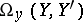, where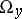is the curvature form at the point, whereruns through the set of points which may be connected with the beginning point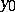by a horizontal path, andand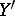are arbitrary horizontal vectors.

How to Cite This Entry:
Holonomy group. Encyclopedia of Mathematics. URL: http://encyclopediaofmath.org/index.php?title=Holonomy_group&oldid=32770
This article was adapted from an original article by G.F. Laptev (originator), which appeared in Encyclopedia of Mathematics - ISBN 1402006098. See original article